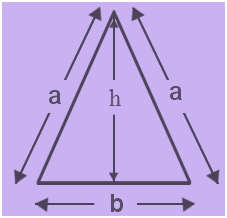Area of Isosceles Triangle Formula

An isosceles triangle is a triangle with two equal sides and has one unequal side. The angles opposite the equal sides are also equal. The equal sides are called legs and the third side is called the base. The angle included by the legs is called the vertex angle and the angles that have the base as one of their sides are called the base angles.$\LARGE Area\;of\;Isoscele\;Triangle\;Formula=\frac{1}{2}bh$

$\LARGE Altitude\;of\;an\;Isosceles\;Triangle=\sqrt{a^{2}-\frac{b^{2}}{4}}$

Where,
b = Base of the isosceles triangle
h = Height of the isosceles triangle &
a = length of the two equal sides

Question 1: Find the area of an isosceles triangle given b = 12 cm and h = 17 cm ?
Solution:
Base of the Triangle (b) = 12 cm
Height of the Triangle (h) = 17 cm
Area of Isosceles Triangle = $\frac{1}{2}$ b $\times$ h

$\frac{1}{2 }$ 12 $\times$ 17

= $\frac{1}{2 }$ $\times$ 204

= 102 cm2

Question 2: Find the length of the base of an isosceles triangle whose area is 243 cm2  and the altitude of the triangle is 27 cm.
Solution:
Area of the Triangle = A = 243 cm2
Height of the Triangle (h) = 27 cm
Base of the Triangle = b = ?

Area of Isosceles Triangle = $\frac{1}{2}$ b $\times$ h

243 = $\frac{1}{2}$ b $\times$ 27

243 = $\frac{b \times 27}{2}$

b = $\frac{243 \times 2}{27}$

b = 18 cm

Thus, the base of the triangle is 18 cm.

Also, check the Isosceles Triangle Perimeter Formula